×

Search anything:

# LBPH algorithm for Face Recognition

#### Machine Learning (ML)Reading time: 30 minutes | Coding time: 10 minutes

In this article, we will explore the Local Binary Patterns Histogram algorithm (LBPH) for face recognition. It is based on local binary operator and is one of the best performing texture descriptor.

The need for facial recognition systems is increasing day by day. They are being used in entrance control, surveillance systems, smartphone unlocking etc. In this article we will use LBPH to extract features from an input test image and match them with the faces in system's database.

## Local Binary Patterns Histogram (LBPH)

Local Binary Patterns Histogram algorithm was proposed in 2006. It is based on local binary operator. It is widely used in facial recognition due to its computational simplicity and discriminative power.

The steps involved to achieve this are:

• creating dataset
• face acquisition
• feature extraction
• classification

The LBPH algorithm is a part of opencv.

## Steps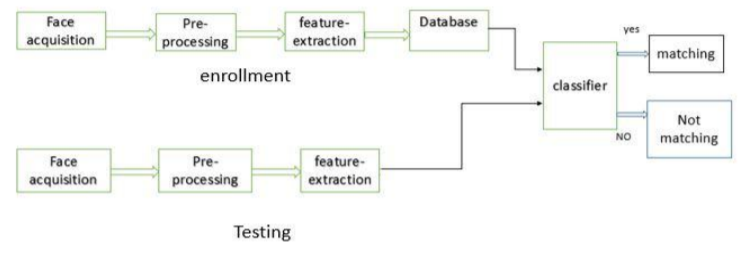• Suppose we have an image having dimentions N x M.
• We divide it into regions of same height and width resulting in m x m dimension for every region.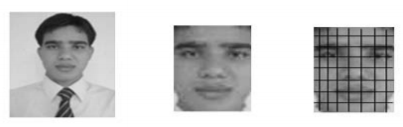• Local binary operator is used for every region. The LBP operator is defined in window of 3x3.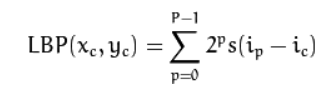here '(Xc,Yc)' is central pixel with intensity 'Ic'. And 'In' being the intensity of the the neighbor pixel

• Using median pixel value as threshold, it compares a pixel to its 8 closest pixels using this function.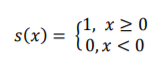• If the value of neighbor is greater than or equal to the central value it is set as 1 otherwise it is set as 0.

• Thus, we obtain a total of 8 binary values from the 8 neighbors.

• After combining these values we get a 8 bit binary number which is translated to decimal number for our convenience.

• This decimal number is called the pixel LBP value and its range is 0-255.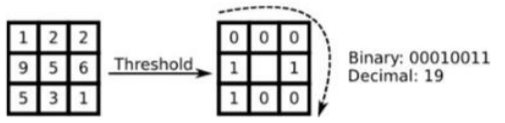• Later it was noted that a fixed neighborhood fails to encode details varying in scale .The algorithm was improved to use different number of radius and neighbors , now it was known as circular LBP.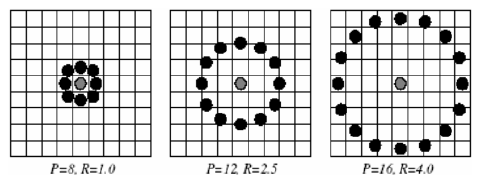• The idea here is to align an arbitrary number of neighbors on a circle with a variable radius. This way the following neighborhoods are captured: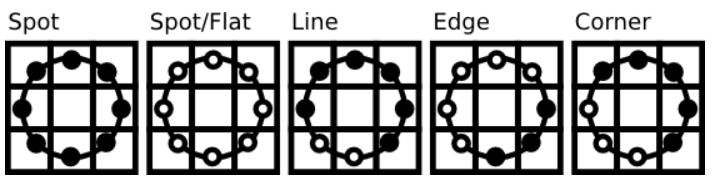• For a given point (Xc,Yc) the position of the neighbor (Xp,Yp), p belonging to P can be calculated by: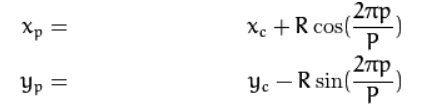here R is radius of the circle and P is the number of sample points.

• If a points coordinate on the circle doesn’t correspond to image coordinates, it get’s interpolated generally by bilinear interpolation: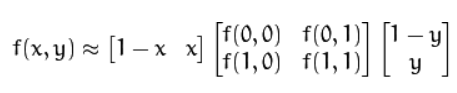• The LBP operator is robust against monotonic gray scale transformations.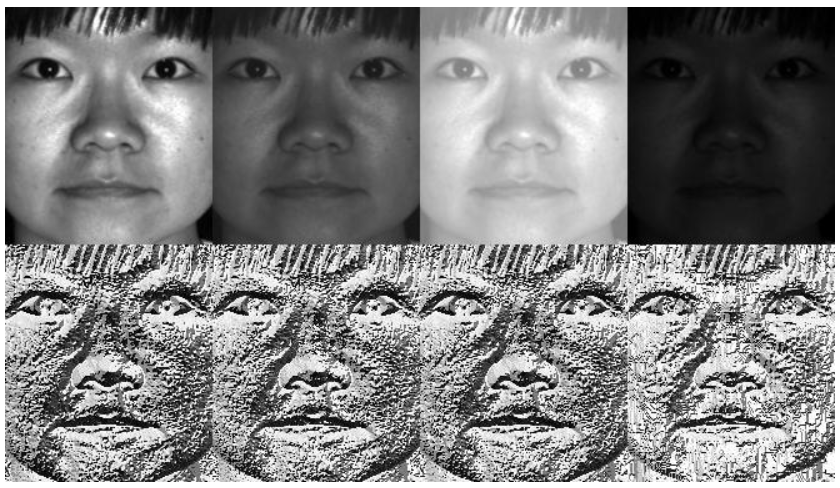• After the generation of LBP value histogram of the region is created by counting the number of similar LBP values in the region.

• After creation of histogram for each region all the histograms are merged to form a single histogram and this is known as feature vector of the image.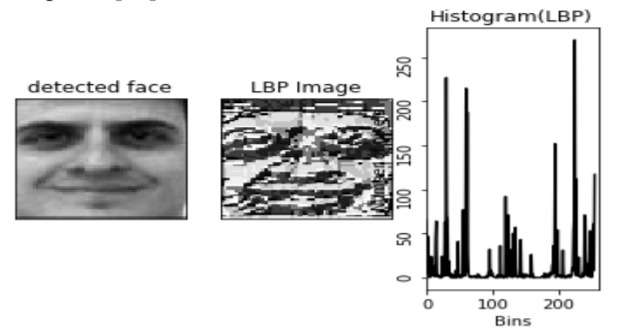• Now we compare the histograms of the test image and the images in the database and then we return the image with the closest hitogram.
( This can be done using many techniques like euclidean distance, chi-square, absolute value etc )

• The Euclidean distance is calculated by comparing the test image features with features stored in the dataset.The minimum distance between test and original image gives the matching rate.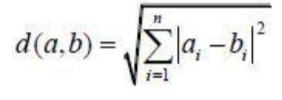• As an output we get an ID of the image from the database if the test image is recognised.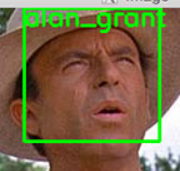LBPH can recognise both side and front faces and it is not affected by illumination variations which means that it is more flexible.

## Implementation

• The dataset can be created by taking images from webcam or from saved images. We will take many samples of a single person. An unique ID or a name is given to a person in the database.
``````# Import the necessary libraries
import numpy as np
import cv2
import os
``````
• Creating database by adding names of peopple we wanna add to database
``````# creating database
database = ["Tom Cruise", "Clinton"]
``````
• Face detection using Haarcascade Classifier, cropping the face and grayscaling the face .
``````def face_detection(image):
image_gray = cv2.cvtColor(image, cv2.COLOR_BGR2GRAY)
face = haar_classifier.detectMultiScale(image_gray, scaleFactor=1.3, minNeighbors=7)
(x,y,w,h) = face
return image_gray[y:y+w, x:x+h], face
``````
• Preparing data with labels and faces
``````def prepare_data(data_path):
folders = os.listdir(data_path)
labels = []
faces = []
for folder in folders:
label = int(folder)
training_images_path = data_path + '/' + folder
for image in os.listdir(training_images_path):
image_path = training_images_path + '/' + image
face, bounding_box = face_detection(training_image)
faces.append(face)
labels.append(label)

print ('Training Done')
return faces, labels
``````
``````faces, labels = prepare_data('training')
print ('Total faces = ', len(faces))
print ('Total labels = ', len(labels))
``````
• Creating LBPH model and training it with the prepared data
``````model = cv2.face.createLBPHFaceRecognizer()
model.train(faces, np.array(labels))
``````
• Testing the trained model using a test image
``````def predict_image(test_image):
img = test_image.copy()
face, bounding_box = face_detection(img)
label = model.predict(face)
label_text = database[label-1]
print (label)
print (label_text)
(x,y,w,h) = bounding_box
cv2.rectangle(img, (x,y), (x+w, y+h), (0,255,0), 2)
cv2.putText(img, label_text, (x,y), cv2.FONT_HERSHEY_PLAIN, 1.5, (0, 255, 0), 2)
return img
``````
``````test1 = cv2.imread("test/tom.jpg")
predict1 = predict_image(test1)
cv2.imshow('Face Recognition', predict1)
cv2.waitKey(0)
cv2.destroyAllWindows()
``````

• LBPH Method is one of the best performing texture descriptor.
• The LBP operator is robust against monotonic gray scale transformations.
• FisherFaces only prevents features of a person from becoming dominant, but it still considers illumination variations as a useful feature. But light variation is not a useful feature to extract as it is not part of the actual face.
• Fisherfaces need larger storage of face data and more processing time in recognition.
• In LBPH each image is analyzed independently, while the eigenfaces and fisherfaces method looks at the dataset as a whole.
• LBPH method will probably work better than fisherfaces in different environments and light conditions .It also depends on our training and testing data sets.
• It can represent local features in the images.
• LBPH can recognise both side and front faces.

## Conclusion

• Computer vision is a major part of every big project relates to security, banking, marketing etc.
• LBHP algorithm can be use in various applications for facial recognition.
• It is very easy to implement.#### Yashwant Saini

engineer.

LBPH algorithm for Face Recognition